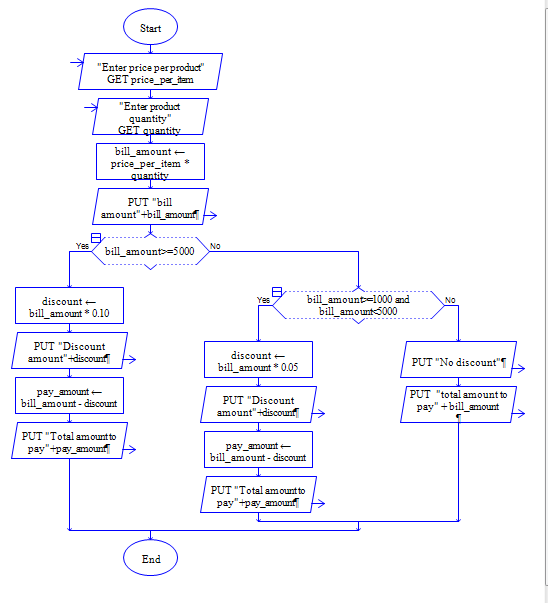# C Program to calculate different discount for different Bill amount

Get price and quantity of a product, calculate bill amount, calculate 10% discount for the bill amount 5000 and above, 5% discount for the bill amount 1000 and above.

Sample Input 1:

10 400

Sample Output 1:

3800

Sample Input 2:

10 500

Sample Output 2:

4500

#### Flow Chart Design#### Program or Solution

``` #include <stdio.h> int main(void) { double quantity,price,amount,discount; printf("Enter quantity and price:"); scanf("%lf %lf",&quantity, &price); amount=quantity*price; if(amount>5000) { discount=amount*0.10; } else if(amount > 1000) { discount=amount*0.05; } else { discount=0; } amount = amount - discount; printf("%lf",amount); return 0; } ```

#### Program Explanation

chained conditional check is used here to check whether amount is greater than 1000 or greater than 5000.

any one of the expression to calculate discount will be executed.